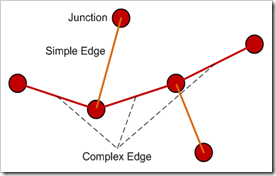## Geometric Networks in ArcGIS

When modelling networks in ArcGIS there are 2 basic options you can choose:
• Network datasets
• Geometric networks
Network datasets are good for modelling undirected networks because they can allow flow in any direction. Network datasets are based on junctions, edges, and turn sources, which are simple feature classes. Network datasets are best suited for modelling transportation networks such as roads. Given that the work I do is not really concerned with road networks I’m going to put network datasets on the back burner.
Geometric datasets are better suited to modelling utilities (e.g. gas or oil pipelines), and natural resources (e.g. river systems). This is of much greater interest to me at the moment, hence this post. In essence a geometric network is a set of features that form a connected system of edges and junctions. An important characteristic of geometric networks is that features are regarded as being connected if they exist at the same x,y coordinate (geometric coincidence).
So, geometric networks are based on 2 main elements: edges and junctions.

#### Edges

Edges are features that have a length and through which some commodity flows.
There are 2 types of edge:
• Simple – A line between two adjacent junctions. Resources enter one end of an edge and exit at the other end.
• Complex – A set of connected lines with 2 or more junctions. Resources flow from one end to another but may be ‘siphoned off’ along the edge without having to split the edge feature.Note that in the diagram above the complex edge is intersected by 2 simple edges but it is not split by them. The complex edge is one continuous feature. This could represent a gas main (complex edge) intersected by service mains (simple edges).
##### Connectivity
Connectivity for simple edges occurs only at the end points of the feature. Connectivity for complex edges occurs at the ends of features but also at mid-span intersections.
Connections between coincident junctions is not permitted. You can only connect junctions through edges.
You can specify rules to constrain connectivity between features. For example you can specify edge-junctions rules to stipulate that only edges of a certain type can connect to junctions of a certain type. Similarly you can specify edge-edge rules to constrain what types of edge can connect to each other.

#### Junctions

Junctions represent the the location of endpoints of edges or where multiple edges are connected. A junction can connect 2 or more edges and facilitates flow of a commodity (traffic, water, etc.) from one edge to another.

#### Logical network

A geometric network will have a corresponding logical network. The logical is not concerned with coordinate values but rather data about how the network is connected (connectivity, weights, and other information). The logical network is a graph stored as tables in the geodatabase and is used for tracing and flow operations. This is primarily acheived through the use of a connectivity table that lists, for every junction, adjacent junctions and edges.

#### Sources and Sinks

Any of the features in the geometric dataset can take on the role of a source (where a commodity flows from) or a sink (where a commodity terminates). These roles are used to define flow in the network.

#### Weights

Features can have a weight which represents the cost of traversing the edge or passing through a junction. Any number of attributes can be used as weights, for example lengths, capacity or slope.
Friday, 8 April 2011